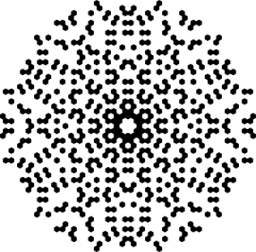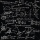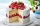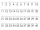# Common divisors

Find all common divisors of numbers 30 and 45.

Result

d = (Correct answer is: 1, 3, 5, 15)#### Solution:We would be very happy if you find an error in the example, spelling mistakes, or inaccuracies, and please send it to us. We thank you!Tips to related online calculators
Do you want to calculate greatest common divisor two or more numbers?

## Next similar math problems:

• SchoolLess than 500 pupils attend school. When it is sorted into pairs, one pupil remains. Similarly, when sorted into 3, 4, 5 and 6 members team one remains. Sorted to seven members teams, no left behind. How many pupils are attending this school?
• TogetherIf 8 men, 10 women, 16 children collects ₹1024 in 4 days, how many days will be required for 6 men, 5 women and 4 boys to collect ₹768? (₹ is Indian Rupee)
• Year 2020The four-digit number divided by 2020 gives a result of 1, **. (Can not be in form 1,*0. ) Write all the options.
• Closest natural numberFind the closest natural number to 4.456 to 44.56 and to 445.6.
• Positive integersSeveral positive integers are written on the paper. Michaella only remembered that each number was half the sum of all the other numbers. How many numbers could be written on paper?
• Candy and boxesWe have some number of candy and empty boxes. When we put candies in boxes of ten, there will be 2 candies and 8 empty boxes left, when of eight, there will be 6 candies and 3 boxes left. How many candy and empty boxes left when we put candies in boxes of
• TrianglesIvo wants to draw all the triangles whose two sides of which have a length of 4 cm and 9 cm and the length of the third side is expressed in whole centimeters. How many triangles does he have?
• Year 2018The product of the three positive numbers is 2018. What are the numbers?
• DozenWhat is the product of 26 and 5? Write the answer in Arabic numeral. Add up the digits. How many of this is in a dozen? Divide #114 by this
• Collection of stampsJano, Rado, and Fero have created a collection of stamps in a ratio of 5: 6: 9. Two of them had 429 stamps together. How many stamps did their shared collection have?
• My fatherMy father has a big farm. 6/8 of it were planted with mango trees, 1/2 of the remainder are guava trees and the rest 10 trees are santol trees. What is the number of all trees?
• Quarantine cupcakesMr. Honse was baking quarantine cupcakes. Mrs. Carr made twice as many as Mr. Honse. Ms. Sanchez made 12 cupcakes more than Mr. Honse. If they put all their cupcakes together (which they can’t because. .. quarantine!) they would have 108 cupcakes. How may
• BoatsThree-quarters of boats are white, 1/7 are blue and 9 are red. How many boats do we have?
• Progression-12, 60, -300,1500 need next 2 numbers of pattern
• Tenths digitFor 10.932, which digit is in the tenths place?
• The temperatureThe temperature at 1:00 was 10 F. Between 1:00 and 2:00, the temperature dropped 15F. Between 2:00 and 3:00, the temperature rose 3F. What is the temperature at 3:00?
• Expression 6Evaluate expression: -6-2(4-8)-9